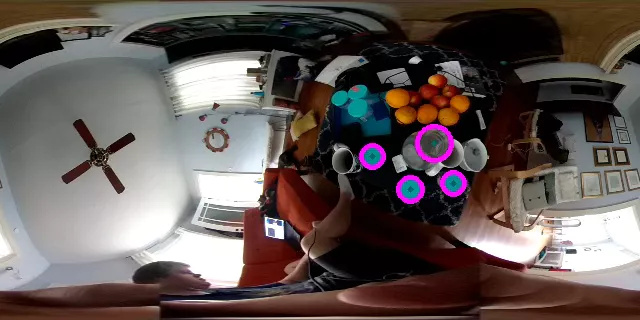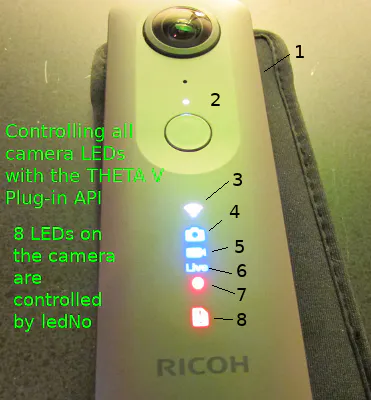# Circle Detector by Alex Merchen

## Get Plug-in From the THETA Plug-in Store

This article was written by Alex Merchen.

Create an plug-in that will be able to detect circles in 360 degree images.

## Story

### How to Use/Proof it works

The goal of this project it to use the Ricoh Theta V to identify circles in real time. The Ricoh Theta V has built in android and you can develop it in Android Studio. To launch the plug-in, I used Vysor to show the android menu so I could watch in real time this working:

1 / 5 • Launch it in the Vysor app

Just to show an instance where this works well:

Original image, I put some cups on a tableIdentified four of them and drew circles around them.

### How to Build

So I wanted my plug-in to do a few things with the most important ones being to identify circles and the second to be save them. I want to be able to count circles because that will allow me to eventually count people and save pictures of when there are too many people. I also decided I wanted to be able to tell on the device itself when the device was taking pictures instead of blindly guessing that it was working correctly.

I started with the easier of the goals - the LED changing colors when something is happening. Ricoh Theta has a tutorial that outlines changing LED colors : http://theta360.guide/plugin-guide/tutorialcolor/

That tutorial is pretty good but the summary for me was doing the following:

``````notificationLedHide(LedTarget.LED4);takePicture(dateTimeStr);saveProcessWindow(mOutputFrame, dateTimeStr);notificationLedShow(LedTarget.LED4);
``````

I made it so that the LED4 (the camera icon) turn off when I was about to take a picture and then turn back on when it was finished processing. This allowed the camera to have a flickering effect when it was working which made it significantly easier to tell that it was working correctly. Those two lines effectively solve the “I don’t know if this is working correctly” problem.The LEDs available - this is in Ricoh Theta’s tutorial on LEDs

The next step in it is to identify the circles. Essentially, I take in an image that the camera sees, I turn it transform it into a gray image (line 2). From there, I put a little bit of a blur on it and I use the org.opencv.imgproc.Imgproc function HoughCircles to determine if there are any circles in the area. There is a lot of very helpful information about opencv on their website: https://docs.opencv.org/3.4/index.html. I could look at all of the circles in the image and add a circle around the center and add a center to the circle. This is added to my mOutputFrame which will then be saved later.

``````Mat gray = new Mat();Imgproc.cvtColor(inputFrame.rgba(), gray, Imgproc.COLOR_BGR2GRAY);Imgproc.medianBlur(gray,gray, 5);Mat circles = new Mat();Imgproc.HoughCircles(gray, circles, Imgproc.HOUGH_GRADIENT, 1.0, (double)gray.rows()/16, 100.0, 30.0, 10, 30);mOutputFrame = inputFrame.rgba();for (int x = 0; x < circles.cols(); x ++){    double[] c = circles.get(0,x);    Point center = new Point(Math.round(c), Math.round(c));    Imgproc.circle(mOutputFrame, center, 1, new Scalar(0,100,100), 3, 8, 0 );    // circle outline    int radius = (int) Math.round(c);    Imgproc.circle(mOutputFrame, center, radius, new Scalar(255,0,255), 3, 8, 0 );}
``````

I started with the theta-skunkworks’s opencv detection program and modified the code with the changes shown above - https://github.com/theta-skunkworks/theta-plugin-opencv-detection-sample

In the MainActivity under java, I modified the onCameraFrame public function:

``````public Mat onCameraFrame(CvCameraViewFrame inputFrame) {        Mat gray = new Mat();        Imgproc.cvtColor(inputFrame.rgba(), gray, Imgproc.COLOR_BGR2GRAY);        Imgproc.medianBlur(gray,gray, 5);        Mat circles = new Mat();        Imgproc.HoughCircles(gray, circles, Imgproc.HOUGH_GRADIENT, 1.0, (double)gray.rows()/16, 100.0, 30.0, 10, 30);        mOutputFrame = inputFrame.rgba();        for (int x = 0; x < circles.cols(); x ++){            double[] c = circles.get(0,x);            Point center = new Point(Math.round(c), Math.round(c));            Imgproc.circle(mOutputFrame, center, 1, new Scalar(0,100,100), 3, 8, 0 );            int radius = (int) Math.round(c);            Imgproc.circle(mOutputFrame, center, radius, new Scalar(255,0,255), 3, 8, 0 );        }        if (canProcess()) {            if (circles.cols()>0) {                mStartProcessingTime = System.currentTimeMillis();                String dateTimeStr = getDateTimeStr();                notificationLedHide(LedTarget.LED4);                takePicture(dateTimeStr);                saveProcessWindow(mOutputFrame, dateTimeStr);                notificationLedShow(LedTarget.LED4);            }        }        return mOutputFrame;    }
``````